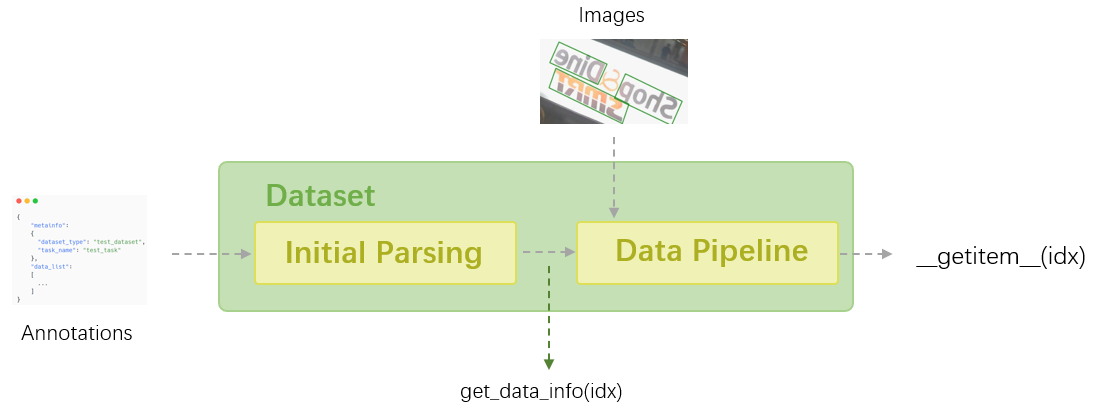Shortcuts

# 数据集类¶

## 概览¶Dataset 类支持一些高级功能，例如懒加载、数据序列化、利用各种数据集包装器执行数据连接、重复和类别平衡。这些内容将不在本教程中介绍，但您可以阅读 MMEngine: BaseDataset 了解更多详细信息。

## 常见接口¶

from mmocr.datasets import OCRDataset
from mmengine.registry import init_default_scope
init_default_scope('mmocr')

train_pipeline = [
dict(
dict(
with_polygon=True,
with_bbox=True,
with_label=True,
),
dict(type='RandomCrop', min_side_ratio=0.1),
dict(type='Resize', scale=(640, 640), keep_ratio=True),
dict(
type='PackTextDetInputs',
meta_keys=('img_path', 'ori_shape', 'img_shape'))
]
dataset = OCRDataset(
data_root='tests/data/det_toy_dataset',
ann_file='textdet_test.json',
test_mode=False,
pipeline=train_pipeline)



>>> print(len(dataset))

10


>>> from pprint import pprint
>>> pprint(dataset.metainfo)

{'category': [{'id': 0, 'name': 'text'}],
'dataset_type': 'TextDetDataset',


>>> from pprint import pprint
>>> pprint(dataset.get_data_info(0))

{'height': 720,
'img_path': 'tests/data/det_toy_dataset/test/img_10.jpg',
'instances': [{'bbox': [260.0, 138.0, 284.0, 158.0],
'bbox_label': 0,
'ignore': True,
'polygon': [261, 138, 284, 140, 279, 158, 260, 158]},
...,
{'bbox': [1011.0, 157.0, 1079.0, 173.0],
'bbox_label': 0,
'ignore': True,
'polygon': [1011, 157, 1079, 160, 1076, 173, 1011, 170]}],
'sample_idx': 0,
'seg_map': 'test/gt_img_10.txt',
'width': 1280}


• inputs：经过数据增强后的图像；

• data_samples：包含经过数据增强后的标注和元信息的 DataSample，这些元信息可能由一些数据变换产生，并用以记录该样本的某些关键属性。

>>> pprint(dataset)

{'data_samples': <TextDetDataSample(

META INFORMATION
ori_shape: (720, 1280)
img_path: 'tests/data/det_toy_dataset/imgs/test/img_10.jpg'
img_shape: (640, 640)

DATA FIELDS
gt_instances: <InstanceData(

META INFORMATION

DATA FIELDS
labels: tensor([0, 0, 0])
polygons: [array([207.33984 , 104.65409 , 208.34634 ,  84.528305, 231.49594 ,
86.54088 , 226.46341 , 104.65409 , 207.33984 , 104.65409 ],
dtype=float32), array([237.53496 , 103.6478  , 235.52196 ,  84.528305, 365.36096 ,
86.54088 , 364.35446 , 107.67296 , 237.53496 , 103.6478  ],
dtype=float32), array([105.68293, 166.03773, 105.68293, 151.94969, 177.14471, 150.94339,
178.15121, 165.03145, 105.68293, 166.03773], dtype=float32)]
ignored: tensor([ True, False,  True])
bboxes: tensor([[207.3398,  84.5283, 231.4959, 104.6541],
[235.5220,  84.5283, 365.3610, 107.6730],
[105.6829, 150.9434, 178.1512, 166.0377]])
) at 0x7f7359f04fa0>
) at 0x7f735a0508e0>,
'inputs': tensor([[[129, 111, 131,  ...,   0,   0,   0], ...
[ 19,  18,  15,  ...,   0,   0,   0]]], dtype=torch.uint8)}


## 数据集类及标注格式¶

### OCRDataset¶

#### 标注格式¶

{
"metainfo":
{
"dataset_type": "TextDetDataset",  # 可选项: TextDetDataset/TextRecogDataset/TextSpotterDataset
"category": [{"id": 0, "name": "text"}]  # 在 textdet/textspotter 里用到
},
"data_list":
[
{
"img_path": "test_img.jpg",
"height": 604,
"width": 640,
"instances":  # 一图内的多个实例
[
{
"bbox": [0, 0, 10, 20],  # textdet/textspotter 内用到, [x1, y1, x2, y2]。
"bbox_label": 0,  # 对象类别, 在 MMOCR 中恒为 0 (文本)
"polygon": [0, 0, 0, 10, 10, 20, 20, 0], # textdet/textspotter 内用到。 [x1, y1, x2, y2, ....]
"text": "mmocr",  # textspotter/textrecog 内用到
"ignore": False # textspotter/textdet 内用到，决定是否在训练时忽略该实例
},
#...
],
}
#... 多图片
]
}


#### 示例配置¶

pipeline = [
dict(
dict(
with_polygon=True,
with_bbox=True,
with_label=True,
),
dict(
type='PackTextDetInputs',
meta_keys=('img_path', 'ori_shape', 'img_shape'))
]

icdar2015_textdet_train = dict(
type='OCRDataset',
data_root='data/icdar2015',
ann_file='textdet_train.json',
filter_cfg=dict(filter_empty_gt=True, min_size=32),
pipeline=pipeline)

batch_size=16,
num_workers=8,
persistent_workers=True,
sampler=dict(type='DefaultSampler', shuffle=True),
dataset=icdar2015_textdet_train)


### RecogLMDBDataset¶

#### 标注格式¶

MMOCR 会读取 lmdb 数据集中的以下键：

• num_samples：描述数据集的数据量的参数。

• 图像和标签的键分别以 image-000000001label-000000001 的格式命名，索引从1开始。

MMOCR 在 tests/data/rec_toy_dataset/imgs.lmdb 中提供了一个 toy lmdb 数据集。您可以使用以下代码片段了解其格式。

>>> import lmdb
>>>
>>> env = lmdb.open('tests/data/rec_toy_dataset/imgs.lmdb')
>>> txn = env.begin()
>>> for k, v in txn.cursor():
>>>     print(k, v)

b'image-000000001' b'\xff...'
b'image-000000002' b'\xff...'
b'image-000000003' b'\xff...'
b'image-000000004' b'\xff...'
b'image-000000005' b'\xff...'
b'image-000000006' b'\xff...'
b'image-000000007' b'\xff...'
b'image-000000008' b'\xff...'
b'image-000000009' b'\xff...'
b'image-000000010' b'\xff...'
b'label-000000001' b'GRAND'
b'label-000000002' b'HOTEL'
b'label-000000003' b'HOTEL'
b'label-000000004' b'PACIFIC'
b'label-000000005' b'03/09/2009'
b'label-000000006' b'ANING'
b'label-000000007' b'Virgin'
b'label-000000008' b'america'
b'label-000000009' b'ATTACK'
b'label-000000010' b'DAVIDSON'
b'num-samples' b'10'



#### 示例配置¶

pipeline = [
dict(
dict(
with_text=True,
),
dict(
type='PackTextRecogInputs',
meta_keys=('img_path', 'ori_shape', 'img_shape'))
]

toy_textrecog_train = dict(
type='RecogLMDBDataset',
data_root='tests/data/rec_toy_dataset/',
ann_file='imgs.lmdb',
pipeline=pipeline)

batch_size=16,
num_workers=8,
persistent_workers=True,
sampler=dict(type='DefaultSampler', shuffle=True),
dataset=toy_textrecog_train)


### RecogTextDataset¶

#### 标注格式¶

img1.jpg OpenMMLab
img2.jpg MMOCR


jsonl 格式使用类似字典的结构来表示标注，其中键 filenametext 存储图像名称和单词标签。

{"filename": "img1.jpg", "text": "OpenMMLab"}
{"filename": "img2.jpg", "text": "MMOCR"}


#### 示例配置¶

pipeline = [
dict(
dict(
with_polygon=True,
with_bbox=True,
with_label=True,
),
dict(
type='PackTextDetInputs',
meta_keys=('img_path', 'ori_shape', 'img_shape'))
]

txt_dataset = dict(
type='RecogTextDataset',
data_root=data_root,
ann_file='old_label.txt',
data_prefix=dict(img_path='imgs'),
parser_cfg=dict(
type='LineStrParser',
keys=['filename', 'text'],
keys_idx=[0, 1]),
pipeline=pipeline)

batch_size=16,
num_workers=8,
persistent_workers=True,
sampler=dict(type='DefaultSampler', shuffle=True),
dataset=txt_dataset)

jsonl_dataset = dict(
type='RecogTextDataset',
data_root=data_root,
ann_file='old_label.jsonl',
data_prefix=dict(img_path='imgs'),
parser_cfg=dict(
type='LineJsonParser',
keys=['filename', 'text'],
pipeline=pipeline))

batch_size=16,
num_workers=8,
persistent_workers=True,
sampler=dict(type='DefaultSampler', shuffle=False),
dataset=jsonl_dataset)


### IcdarDataset¶

#### 标注格式¶

{
"images": [
{
"id": 1,
"width": 800,
"height": 600,
"file_name": "test.jpg"
}
],
"annotations": [
{
"id": 1,
"image_id": 1,
"category_id": 1,
"bbox": [0,0,10,10],
"segmentation": [
[0,0,10,0,10,10,0,10]
],
"area": 100,
"iscrowd": 0
}
]
}


#### 配置示例¶

pipeline = [
dict(
dict(
with_polygon=True,
with_bbox=True,
with_label=True,
),
dict(
type='PackTextDetInputs',
meta_keys=('img_path', 'ori_shape', 'img_shape'))
]

icdar2015_textdet_train = dict(
type='IcdarDatasetDataset',
data_root='data/det/icdar2015',
ann_file='instances_training.json',
filter_cfg=dict(filter_empty_gt=True, min_size=32),
pipeline=pipeline)

batch_size=16,
num_workers=8,
persistent_workers=True,
sampler=dict(type='DefaultSampler', shuffle=True),
dataset=icdar2015_textdet_train)


### WildReceiptDataset¶

#### 标注格式¶

// Close Set
{
"file_name": "image_files/Image_16/11/d5de7f2a20751e50b84c747c17a24cd98bed3554.jpeg",
"height": 1200,
"width": 1600,
"annotations":
[
{
"box": [550.0, 190.0, 937.0, 190.0, 937.0, 104.0, 550.0, 104.0],
"text": "SAFEWAY",
"label": 1
},
{
"box": [1048.0, 211.0, 1074.0, 211.0, 1074.0, 196.0, 1048.0, 196.0],
"text": "TM",
"label": 25
}
], //...
}

// Open Set
{
"file_name": "image_files/Image_12/10/845be0dd6f5b04866a2042abd28d558032ef2576.jpeg",
"height": 348,
"width": 348,
"annotations":
[
{
"box": [114.0, 19.0, 230.0, 19.0, 230.0, 1.0, 114.0, 1.0],
"text": "CHOEUN",
"label": 2,
"edge": 1
},
{
"box": [97.0, 35.0, 236.0, 35.0, 236.0, 19.0, 97.0, 19.0],
"text": "KOREANRESTAURANT",
"label": 2,
"edge": 1
}
]
}


#### 配置示例¶

© Copyright 2020-2030, OpenMMLab. Revision b18a09b2.

Built with Sphinx using a theme provided by Read the Docs.
Versions
latest
stable
0.x
dev-1.x